# Multiplicative arithmetic function

(diff) ← Older revision | Latest revision (diff) | Newer revision → (diff)

An arithmetic function of one argument,, satisfying the condition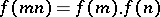(*)

for any pair of coprime integers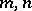. It is usually assumed that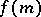is not identically zero (which is equivalent to the condition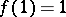). A multiplicative arithmetic function is called strongly multiplicative if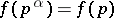for all prime numbersand all natural numbers. If (*) holds for any two numbers, and not just for coprime numbers, thenis called totally multiplicative; in this case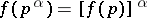.

Examples of multiplicative arithmetic functions. The function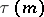, the number of natural divisors of a natural number; the function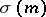, the sum of the natural divisors of the natural number; the Euler function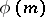; and the Möbius function. The functionis a strongly-multiplicative arithmetic function, a power function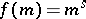is a totally-multiplicative arithmetic function.

The convolution product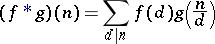yields a group structure on the multiplicative functions. The unit element is given by the function, where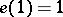andfor all. Another standard multiplicative function is the constant function(for all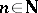) and its inverse, the Möbius function. Note that, wherefor all, and that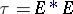,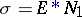.

Formally, the Dirichlet series of a multiplicative functionhas an Euler product: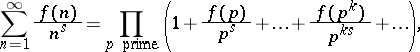whose form simplifies considerably ifis strongly or totally multiplicative.

How to Cite This Entry:
Multiplicative arithmetic function. Encyclopedia of Mathematics. URL: http://encyclopediaofmath.org/index.php?title=Multiplicative_arithmetic_function&oldid=12136
This article was adapted from an original article by I.P. Kubilyus (originator), which appeared in Encyclopedia of Mathematics - ISBN 1402006098. See original article